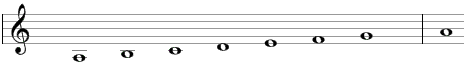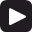# Aeolian scale

The Aeolian scale is a primary heptatonic scale that can be built using the following steps between notes: 1, ½, 1, 1, ½, 1, 1.

One example of the Aeolian scale is A, B, C, D, E, F, G. This example is shown below in traditional notation and in guitar tablature notation.Click Play to hear the Aeolian scale.The Aeolian is the sixth mode of standard major scale or the first mode of the natural minor scale (the natural minor scale itself).

The Aeolian scale is a "primary" heptatonic scale (heptonia prima) as the intervals between any two adjacent notes are either one or two semitones (1 step or ½ steps) and as the two single-semitone intervals are spaced as evenly as possible (going up from the single-semitone interval between B and C we will find two two-semitone intervals - between C and D and D and E – before we get to the second single semitone interval between E and F; going in the opposite direction from the single-semitone interval between B and C we will encounter three two-semitone intervals – between A and B, G and A, and F and G – before we reach again the single-semitone interval between E and F).

## Modes of the Aeolian scale

The following are modes of the Aeolian scale.

1. Aeolian, with steps 1, ½, 1, 1, ½, 1, 1 (A, B, C, D, E, F, G in the example above).
2. Locrian, with steps ½, 1, 1, ½, 1, 1, 1 (B, C, D, E, F, G, A).
3. Ionian or major, with steps 1, 1, ½, 1, 1, 1, ½ (C, D, E, F, G, A, B).
4. Dorian, with steps 1, ½, 1, 1, 1, ½, 1 (D, E, F, G, A, B, C).
5. Phrygian, with steps ½, 1, 1, 1, ½, 1, 1 (E, F, G, A, B, C, D).
6. Lydian, with steps 1, 1, 1, ½, 1, 1, ½ (F, G, A, B, C, D, E).
7. Mixolydian, with steps 1, 1, ½, 1, 1, ½, 1 (G, A, B, C, D, E, F).

## Three-note chords on the Aeolian scale

The following are common triads built on the notes of the Aeolian scale.

• On the root of the scale (on the tonic): minor chord (e.g., Am composed of A, C, E) or suspended chords (Asus4 = A, D, E and Asus2 = A, B, E).
• On the second note (on the supertonic): diminished chord (Bdim = B, D, F).
• On the third note (on the mediant): major chord (C = C, E, G) or suspended chords (Csus4 = C, F, G and Csus2 = C, D, G).
• On the fourth note (on the subdominant): minor chord (Dm = D, F, A) or suspended chords (Dsus4 = D, G, A and Dsus2 = D, E, A).
• On the fifth note (on the dominant): minor chord (Em = E, G, B) or suspended chord (Esus4 = E, A, B).
• On the sixth note (on the submediant): major chord (F = F, A, C) or suspended chord (Fsus2 = F, G, C).
• On the seventh note (on the leading tone, or the subtonic): major chord (G = G, B, D) or suspended chords (Gsus4 = G, C, D and Gsus2 = G, A, D).

## Four-note chords on the Aeolian scale

The following are seventh chords built on the notes of the Aeolian scale.

• On the first note: minor seventh chord (e.g. Amin7 composed of A, C, E, G).
• On the second note: half-diminished seventh chord (minor seventh chord with a flat fifth, Bm7b5 = B, D, F, A).
• On the third note: major seventh chord (Cmaj7 = C, E, G, B).
• On the fourth note: minor seventh chord (Dmin7 = D, F, A, C).
• On the fifth note: minor seventh chord (Emin7 = E, G, B, D).
• On the sixth note: major seventh chord (Fmaj7 = F, A, C, E).
• On the seventh note: dominant seventh chord (G7 = G, B, D, F).

## Intervals on the Aeolian scale

The Aeolian scale is composed of the following intervals.

• A major second, e.g., the interval between A and B is equal to two semitones.
• A minor third, e.g., the interval between A and C is equal to three semitones.
• A perfect fourth, e.g., the interval between A and D is equal to five semitones.
• A perfect fifth, e.g., the interval between A and E is equal to seven semitones.
• A minor sixth, e.g., the interval between A and F is equal to eight semitones.
• A minor seventh, e.g., the interval between A and G is equal to ten semitones.

Scale, Scale (index)

### Filtered HTML

• Freelinking helps you easily create HTML links. Links take the form of [[indicator:target|Title]]. By default (no indicator): Click to view a local node.